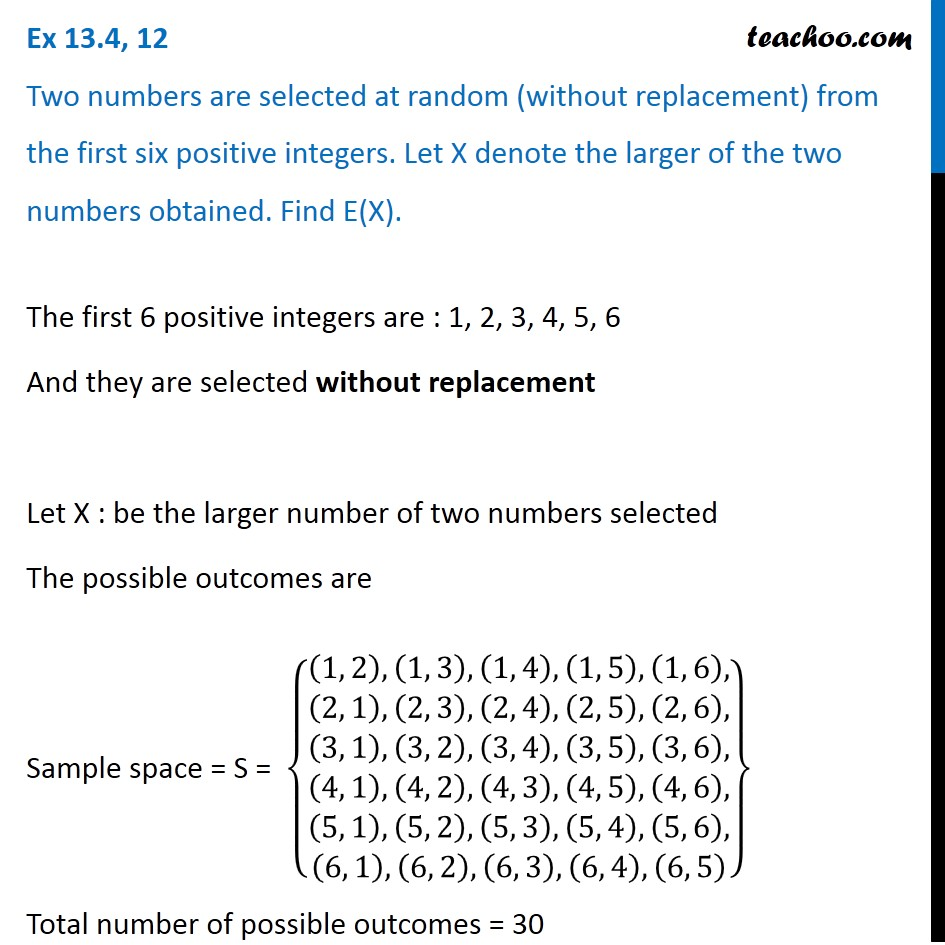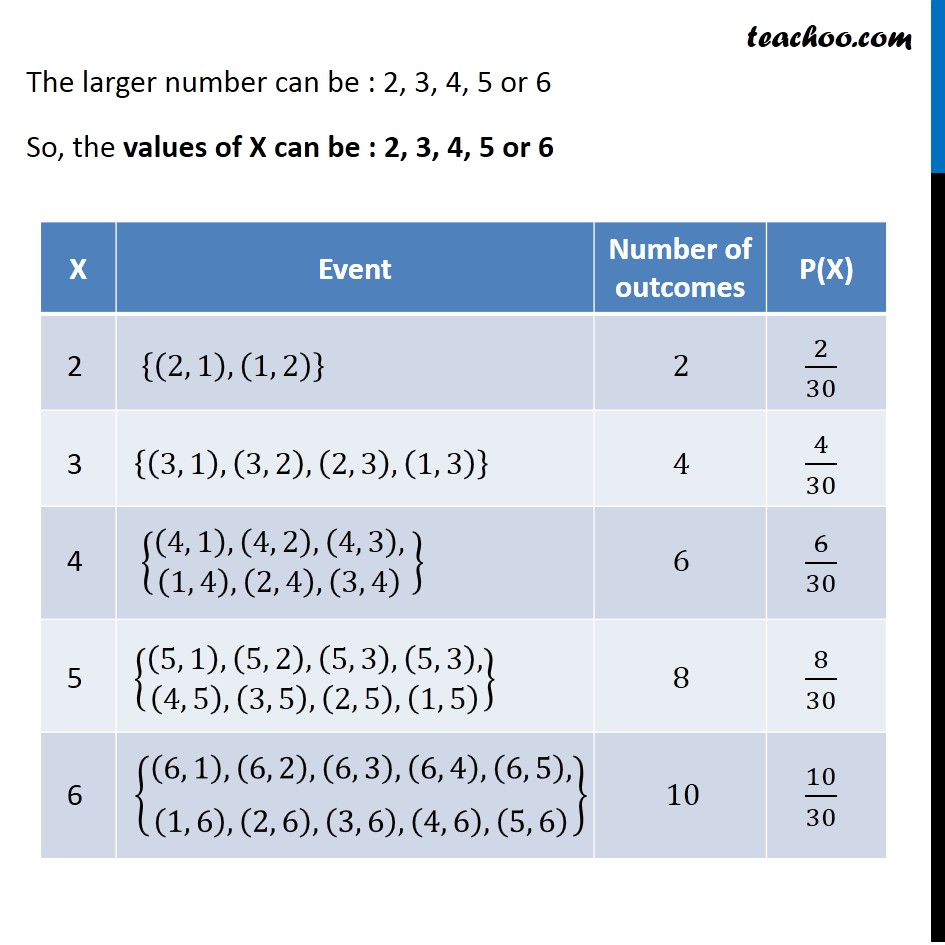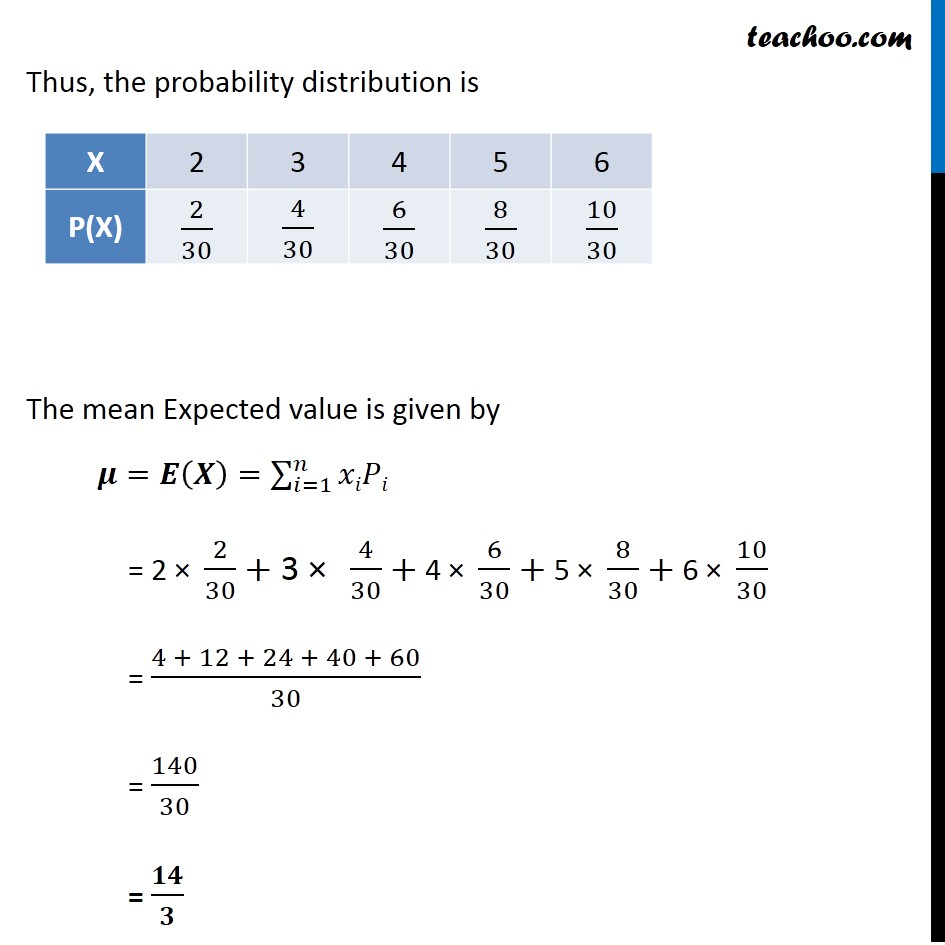Probability Distribution

Chapter 13 Class 12 Probability
Serial order wiseLearn in your speed, with individual attention - Teachoo Maths 1-on-1 Class

### Transcript

Question 12 Two numbers are selected at random (without replacement) from the first six positive integers. Let X denote the larger of the two numbers obtained. Find E(X).The first 6 positive integers are : 1, 2, 3, 4, 5, 6 And they are selected without replacement Let X : be the larger number of two numbers selected The possible outcomes are Sample space = S = {█(█((1, 2),(1, 3),(1, 4),(1, 5),(1, 6),@(2, 1),(2, 3),(2, 4),(2, 5),(2, 6),)@█((3, 1),(3, 2),(3, 4),(3, 5),(3, 6),@(4, 1),(4, 2),(4, 3),(4, 5),(4, 6),)@█((5, 1),(5, 2),(5, 3),(5, 4),(5, 6),@(6, 1),(6, 2),(6, 3),(6, 4),(6, 5) ))} Total number of possible outcomes = 30 The larger number can be : 2, 3, 4, 5 or 6 So, the values of X can be : 2, 3, 4, 5 or 6 Thus, the probability distribution is The mean Expected value is given by 𝝁=𝑬(𝑿)=∑_(𝑖=1)^𝑛▒𝑥𝑖𝑃𝑖 = 2 × 2/30+"3 × " 4/30+ 4 × 6/30+ 5 × 8/30+ 6 × 10/30 = (4 + 12 + 24 + 40 + 60)/30 = 140/30 = 𝟏𝟒/𝟑